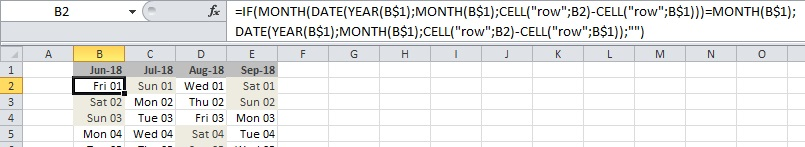# Excel tricks

Written by pmd - - no comments

## 1- Generate a calendar in Excel

Here is how to generate a calendar in Excel. Using Excel 2010 in my case.

In cell B1 (by example), put a date :

`01/06/2018`

In cell B2 :

`=IF(`
```MONTH(DATE(YEAR(B\$1);MONTH(B\$1);CELL("row";B2)-CELL("row";B\$1)))=MONTH(B\$1); DATE(YEAR(B\$1);MONTH(B\$1);CELL("row";B2)-CELL("row";B\$1));"")```

Then you just have to drag-n-drop down and right (or left).

To highlight saturdays and sundays :

`=IF(ISBLANK(B2)=FALSE;OR(IF(WEEKDAY(B2)=7;TRUE;FALSE);IF(WEEKDAY(B2)=1;TRUE;FALSE));FALSE)`

Here is the result :## 2- Number of days in one month

Put a date in cell B1. Then in another cell, formula is :

`=DAY(DATE(YEAR(B1);MONTH(B1)+1;0))`

It will return the number of days of the month in cell B1.